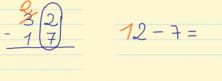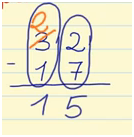Smartick is an advanced online program that teaches kids math and coding in only 15 min. a day

Feb03

# Learn to Subtract with Remainders

Today, we will be learning to use “borrowing,” (sometimes called “regrouping”) in a subtraction problem. In order to do this we need to remember how the elements of a subtraction problem are placed:

• Place the number being subtracted under the number being subtracted from so that place values in the same column match.
• Subtract each column separately starting with the ones.
• Write the result of the subtraction below each column.

Let’s see now how to subtract with remainders:

When the digit being subtracted is greater than the digit being subtracted from, we have to seek help from the top number in the next column (to the left).

For example:
Let’s subtract 32-17.

Place 17 under 32 so that the ones are in the same column, that is, the 7 and 2 coincide.
Start subtracting the ones: 2 – 7, but because 2 is greater than 7, you have to “borrow” from the next column in the top number. A ten is taken from the “30” and given to the 2 in the ones place, to make 12 instead of 2. But since 10 was taken from the 30 (3 in the 10s place), that leaves only 20 (2 in the 10s place).Now you can subtract 12-7 = 5.

Subtract the tens column: 2 – 1 = 1.Therefore, the result of subtraction carried out is 32 – 17 = 15.
I hope this explanation has helped you learn to carry in a subtraction problemIn the following links you will find online exercises to practice subtraction:

If you want to keep learning more elementary math, try Smartick for free!